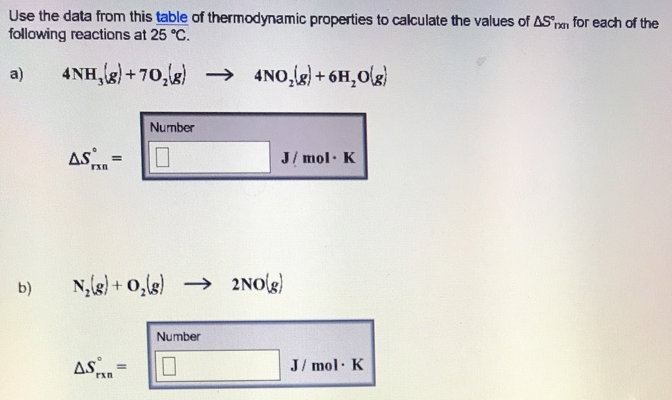# Problem: Use the data from this table of thermodynamic properties to calculate the values of delta S°rxn for each of the following reactions at 25 °C. (a) 4NH3 (g) + 7O2 (g) → 4NO2 (g) + 6H2O (g)      (b) N2 (g) + O2 (g) → 2NO (g)

###### FREE Expert Solution
90% (497 ratings)###### Problem Details

Use the data from this table of thermodynamic properties to calculate the values of delta S°rxn for each of the following reactions at 25 °C.

(a) 4NH3 (g) + 7O2 (g) → 4NO2 (g) + 6H2O (g)

(b) N2 (g) + O2 (g) → 2NO (g)